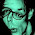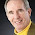## Thursday, April 29, 2010

### A Numerical Poem (Fibonacci)

Consider the following poem involving the Fibonacci numbers:

1/89 = .0        +
.01        +
.001        +
.0002        +
.00003        +
.000005        +
.0000008        +
.00000013        +
.000000021        +
.0000000034        +
.00000000055        +
.000000000089        +
.0000000000144        +
.  ...

What are the characteristics of a (good) poem?  Some insist on beauty, others on truth. Still others like surprise, or layers of meaning uncovered in repeated readings.  Some like concise language, others like patterns--a pattern of sound, or a visual pattern--or maybe, as above, a number pattern.

Mathematicians may assert that good mathematics is poetry--but many of the beautiful and surprising patterns of good mathematics are accessible only to those who have done advanced study. Given above is an example of a mathematical poem:  a sum of decimals that involves the Fibonacci numbers. What qualities make it a poem?  Is this a good poem?   Do you like it?

Recall that the Fibonacci sequence of numbers begins with 0 and 1 and that each succeeding number is the sum of the two preceding.  The first fifteen Fibonacci numbers are: 0, 1, 1, 2, 3, 5, 8, 13, 21, 34, 55, 89, 144, 233, 377.  This poem, with all of its subsequent lines, was proved in 2004 by a Belgian mathematician Johan Claes.  For calculation of a decimal equivalent of 1/89, I used WolframAlpha . After an initial zero the decimal has a sequence of 44 digits that repeat.  Here are the first 60 digits:

1/89 = 0.011235955056179775280898876404494382022471910112359550561797 ...

Our April 19 posting,  "Poems with Fibonacci Number Patterns,"gave links to a number of ways that the Fibonacci numbers occur in nature; this ATT Research link connects to a list of many situations in which the Fibonacci numbers occur in mathematics.  In contrast to "1/89 = . . ." (our numerical poem above) Kathryn DeZur's lines below tell, in a more traditional way, of birth and growth--and remind us once again that patterns in mathematics ofttimes mimic the patterns of life.

Fibonacci Numbers

At a hard wood desk, on a hard
wooden stool, a mathematician counted rings
of monastery bells next door, seeking
God in multiplying numbers. He considered the problem
of ideal fertility: rabbits in fields of buttercups,
daisies, asters, chicory, reproducing at the rate of one
pair per month. The arithmetical series revealed a mean, a golden
number, controlling the growth of leaves, pinecones
tossed in the fire cold winter nights, cauliflower he despised, seeds
that taunted Eve when she delved into the apple
seeking knowledge and gaining children.

I, too, chant the count of hope
each night: one ovum. One sperm. Two eyes. Three-
letter names -- Ann or Ian. Five fingers per hand. A perfect
nautilus spiral of an ear. I long for the fertility
of Fibonacci's numbers, that mystical statistical world
where one plus one equals three.

Kathryn DeZur is an associate professor of English at the State University of New York College of Technology at Delhi. "Fibonacci Numbers" appears in Strange Attractors:  Poems of Love and Mathematics and it first appeared in Literary Mama.

1.2.